# 前言

Pandas的基础数据结构Series和DataFrame。若是还不清楚的可以再去看看我之前的博客详细介绍这两种数据结构的处理方法：

DataFrame行列表查询操作详解+代码实战

DataFrame多表合并拼接函数concat、merge参数详解+代码操作展示

[En]

There are three blogs about null and duplicate values contained in exception values that specifically describe how to deal with them:

1)根据一列分组：

2)根据多列分组

3)组容量

4)遍历

5)多级索引

6）组合操作

1)多聚合

2）重命名

3)多列聚合

4)用户自定义函数

# 数据分组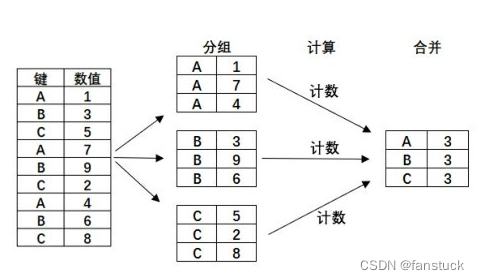## 第一步：分组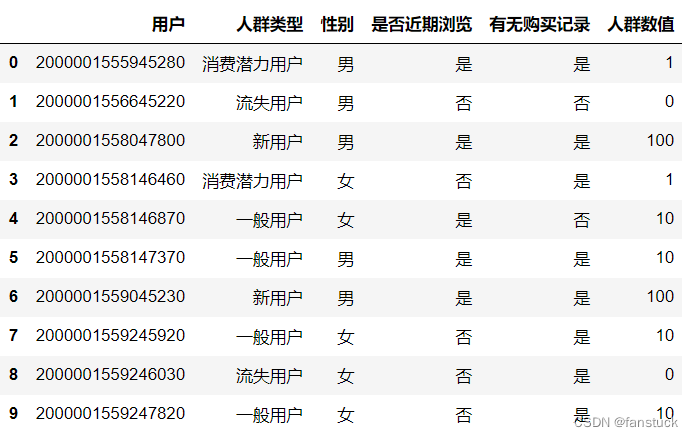``````DataFrame.groupby(by=None, axis=0, level=None, as_index=True, sort=True, group_keys=True, squeeze=False, observed=False, **kwargs)
``````

• by：指定分组的列索引
• axis：0为逐行处理1为逐列处理，默认为0.

• level：若为复合索引则可以调整索引层次

• as_index:聚合输出,返回对象组标签的索引。
• sort：排序
• group_keys：当调用到apply函数时，传入主键
• squeeze:降低维数的返回类型，若为True则仅返回一致的数据类型

``````df1.groupby("人群类型")
``````

``````groupby1=df1.groupby("人群类型")
``````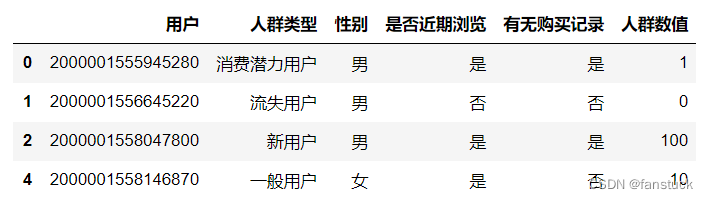``````groupby1.first()
``````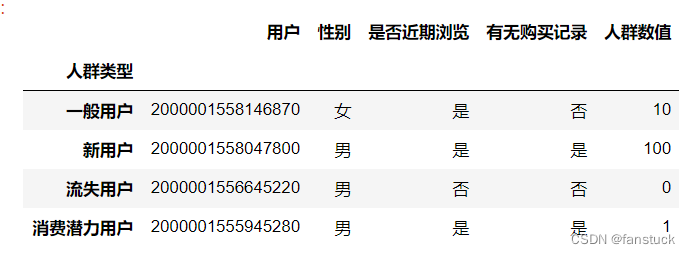### 1)根据一列分组：

``````groupby1=df1.groupby("人群类型")
``````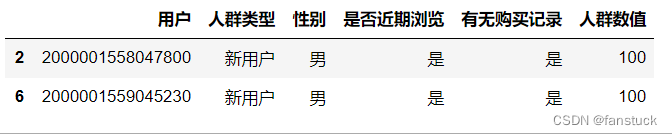### 2)根据多列分组

``````groupby2=df1.groupby(["人群类型","人群数值"])
``````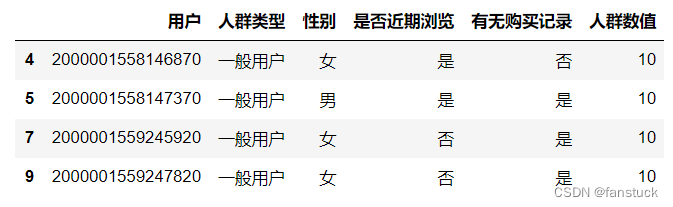### 3)组容量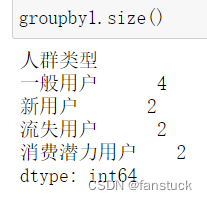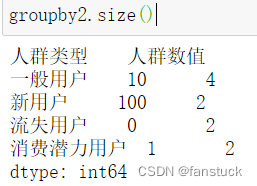### 4)遍历

``````for name,group in grouped_single:
print(name)
``````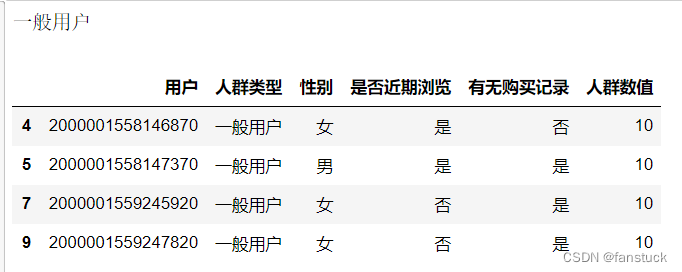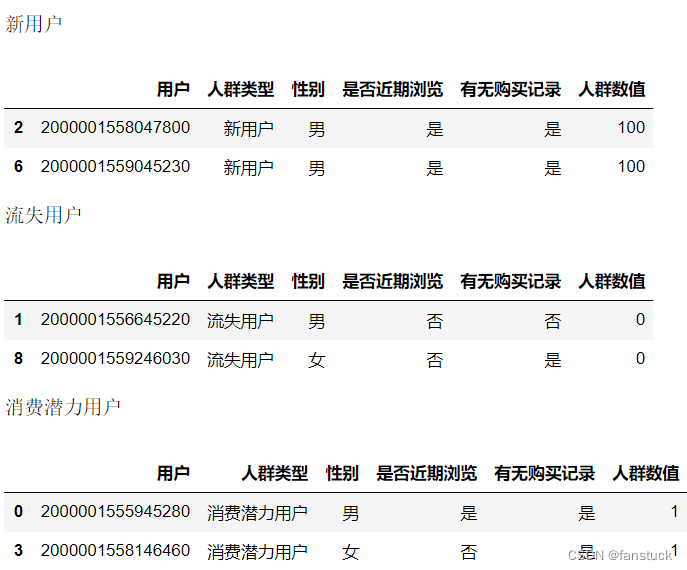### 5)多级索引

``````df2=df1.set_index(['人群类型','性别'])
``````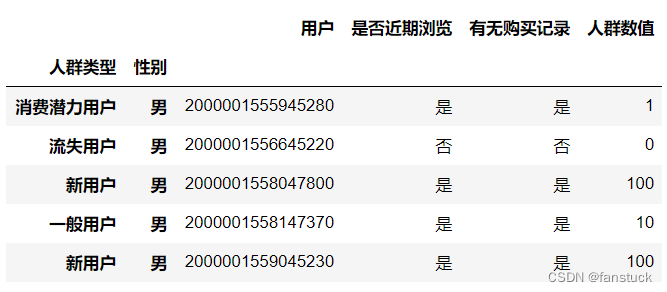### 6）组合操作

``````df1.groupby(['人群类型'])['人群数值'].count()>=3
``````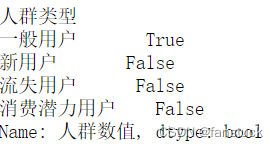``````df1.groupby(['人群类型'])['人群数值'].mean()
``````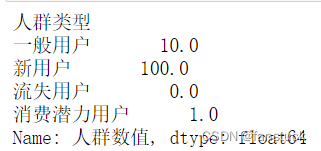## 第二步：聚合

``````DataFrame.agg（func，axis = 0，* args，** kwargs ）
``````

• func：用于聚合数据的函数
• axis：0为逐行操作，1为逐列操作
• args:传递给func的为位置参数
• kwargs：传递给func的关键字参数

``````data = {'name': ['user1', 'user2', 'user3', 'user4', 'user5'],
'old': [21, 21, 21, 19, 26],
'weight': [124, 124, 124,103,121],
'sex':['女','男','男','男','男'],
'species':['一般用户','新用户','一般用户','流失用户','一般用户']}
columns1=['name', 'old', 'weight','sex','species']
index1=['id1', 'id2', 'id3','id4','id5']
df2= pd.DataFrame(data,columns=columns1,index=index1)
``````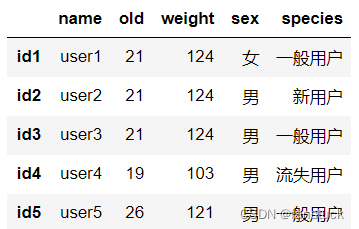### 1)多聚合

``````goupby3=df2.groupby(['species'])
goupby3['old'].agg(['min','mean','max'])
``````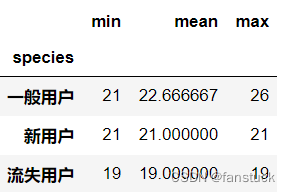### 2）重命名

``````goupby3['old'].agg([('rename_min','min'),('rename_max','max')])
``````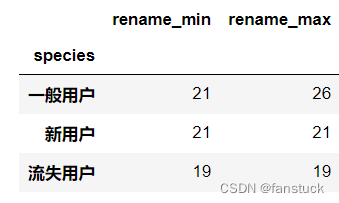### 3)多列聚合

``````goupby3.agg({'old':['min','max'],'weight':['mean']})
``````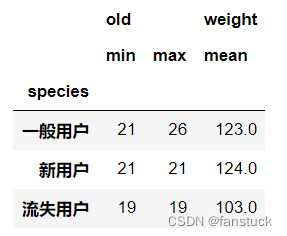### 4)用户自定义函数

agg函数中可嵌套lambda函数：

``````goupby3['old'].agg(lambda x:print(x.head()))
``````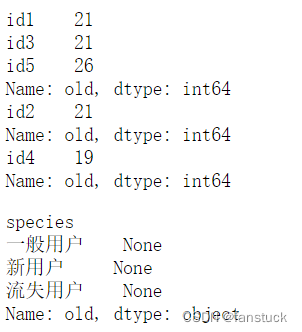``````def f(s,low,high):
return s.between(low,high).max()
goupby3['old'].agg(f,18,20)
``````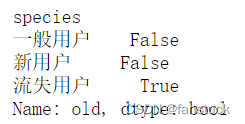## 第三步：apply合并

apply是应用的最广泛的函数，这得益于它的兼容性，我们可以使用apply进行合并分组生成更多我们想要的表格。

``````goupby3.apply(lambda x:x.max())
``````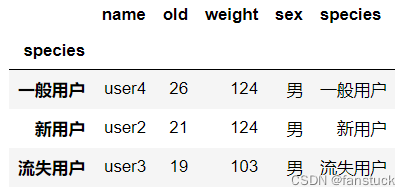``````goupby3['old'].apply(lambda x:x.max()-x.min()).head()
``````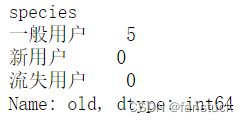# 参阅：

Original: https://blog.csdn.net/master_hunter/article/details/124576184
Author: fanstuck
Title: 一文速学-Pandas数据分组操作方法详解+代码展示

## 1. 打开Anaconda Prompt，查看虚拟环境中安装了那些kernel

``````jupyter kernelspec list
``````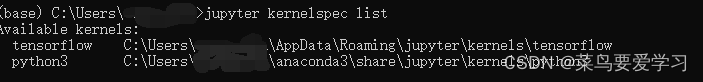## 2.打开Anaconda Prompt，安装ipykernel

``````conda install ipykernel
``````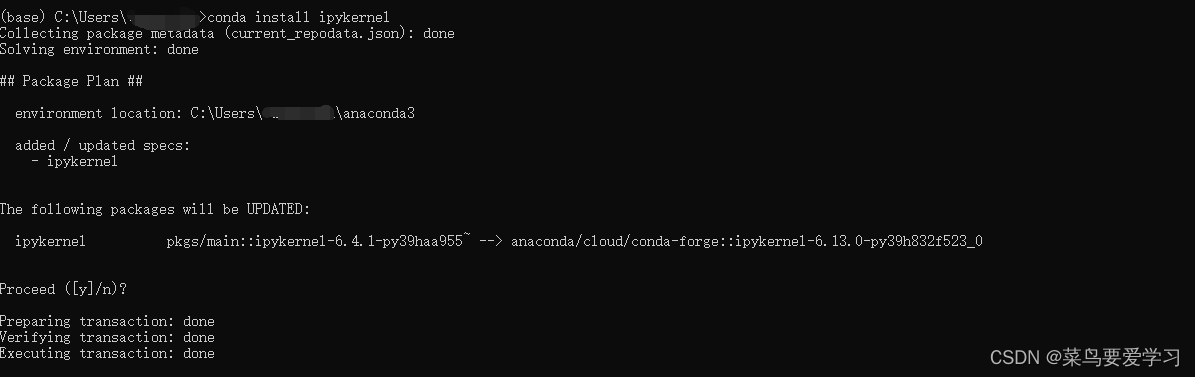## 3.接下来创建ipykernel文件

``````conda install -n 环境名称 ipykernel
``````

``````conda install -n tensorflow ipykernel
``````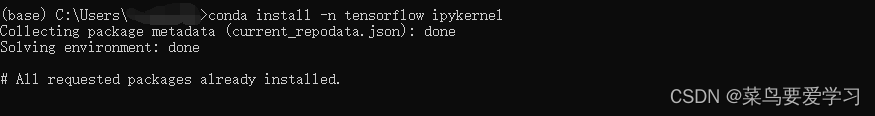## 4.进入我们的GPU环境（首先你要有这个环境）

``````activate 环境名称
``````

``````conda activate tensorflow
``````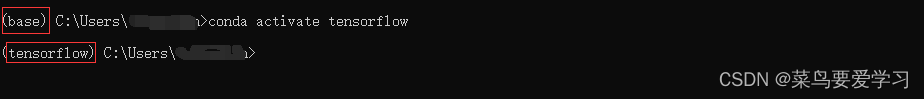## 5.在tensorflow环境中导入kernel

``````python -m ipykernel install --user --name 环境名称
``````

``````python -m ipykernel install --user --name tensorflow
``````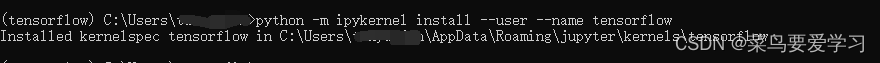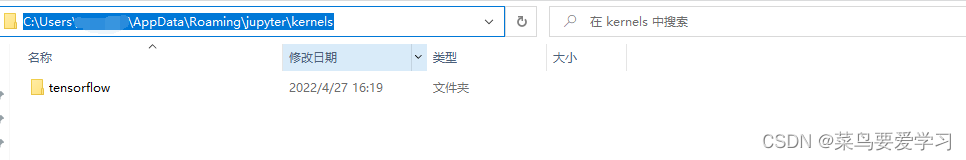## 接下来在桌面打开jupyter notebook，查看是否存在新安装的版本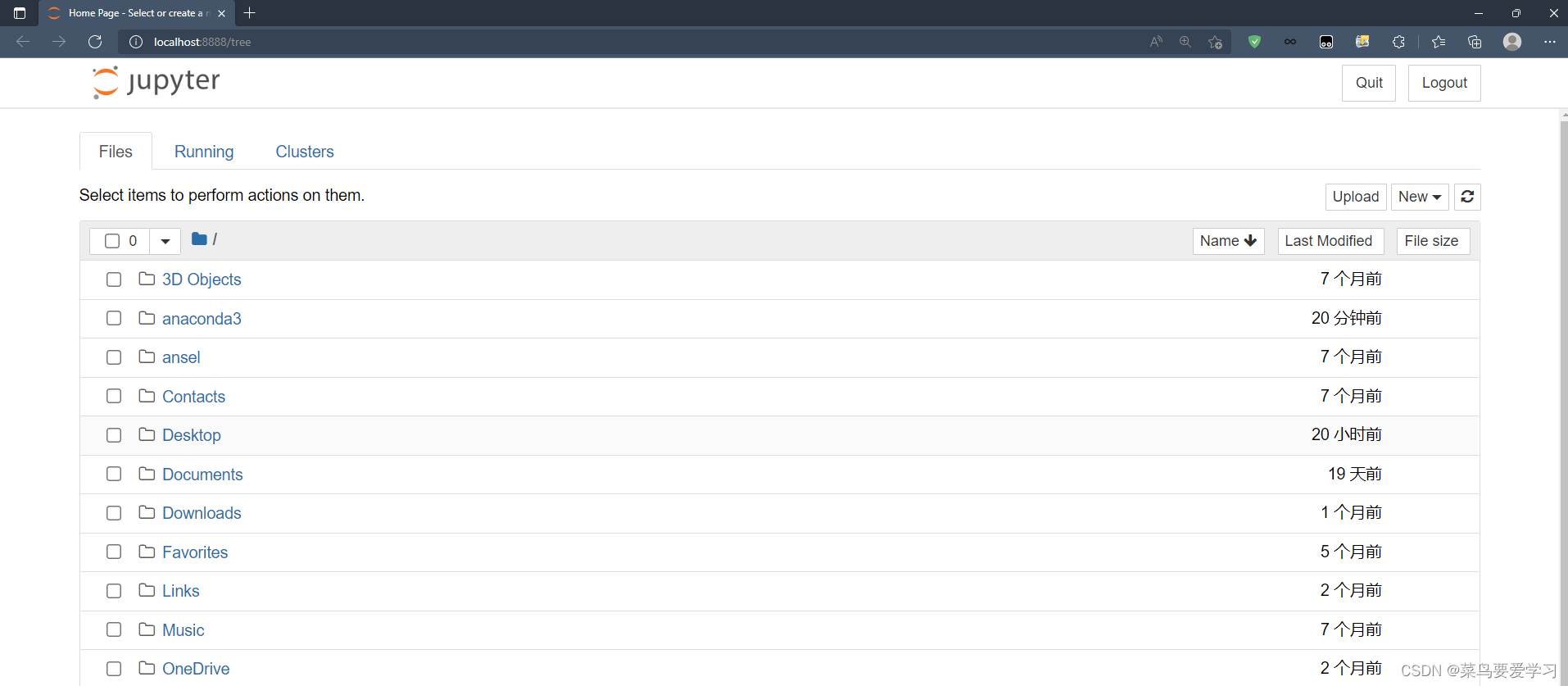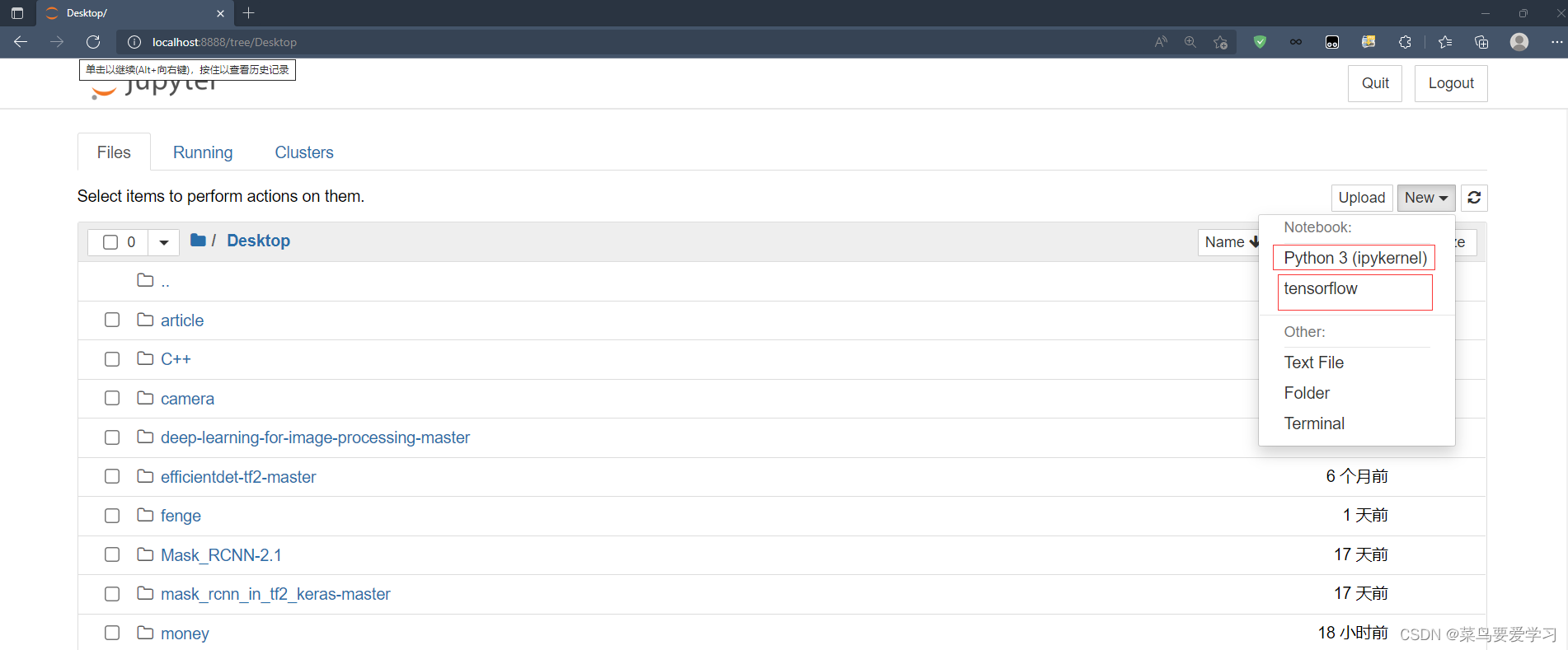``````import tensorflow as tf
import os
os.environ['TF_CPP_MIN_LOG_LEVEL']='2'
a = tf.constant(1.)
b = tf.constant(2.)
print(a+b)
print('GPU:', tf.test.is_gpu_available())
``````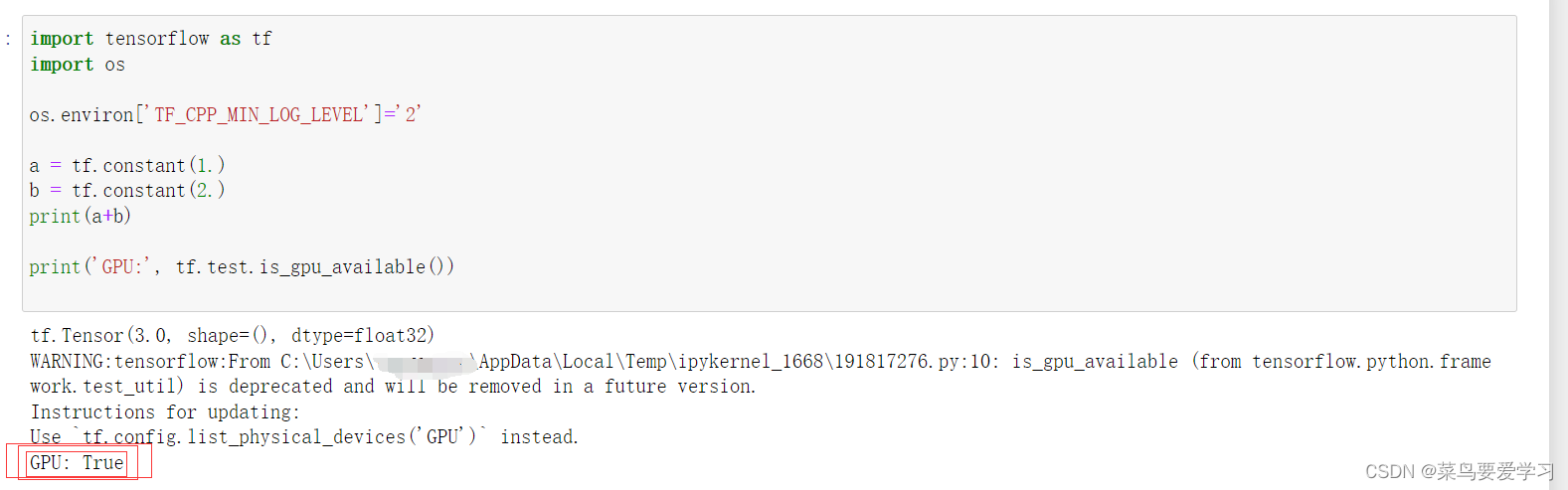Original: https://blog.csdn.net/TYJ00/article/details/124453197
Author: 菜鸟要爱学习
Title: 在jupyter notebook中配置GPU

## 《三英战吕布》 – 图像模板匹配 【Python-Open_CV系列（八）】

《三英战吕布》- 图像模板匹配【Python_Open_CV系列（八）】 文章目录 1.什么是模板匹配？ 及 模板匹配方法matchTemplate() * 介绍 素材准备 2.单模板匹配 * 2.1...

## RGB相机、深度相机以及LiDAR成像原理

RGB相机成像原理 相机结构和原理（入门简版）知乎 摄像机模型数学推导 相机结构 成像原理 大名鼎鼎的"拜尔滤镜" CMOS滤镜排列 像素尺寸、大小关系 景深 景深原理 卷帘相机（rolling sh...